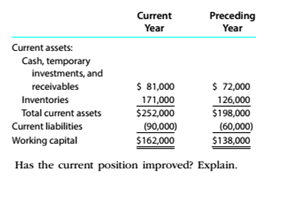Chapter 9, Problem 6CDQ

Chapter
Section
Textbook Problem

For Belzcr Corporation, the working capital at the end of the current year is \$24,000 more than the working capital at the end of the preceding year, reported as follows:To determine

Concept Introduction:

Current Ratio:

Current Ratio is the measure of the company's ability to pay off its current liabilities using its current assets. It is calculated by dividing the total current assets by total current liabilities. The formula of the current ratio is as follows:

Current Ratio=Current assetsCurrent liabilities

To Indicate:

If company's current position has improved.

Explanation

The current ratio of company in the Preceding year is (198000/60000) = 3.3.

The current ratio of company in the Current year is (252000/90000) = 2...

Still sussing out bartleby?

Check out a sample textbook solution.

See a sample solution

The Solution to Your Study Problems

Bartleby provides explanations to thousands of textbook problems written by our experts, many with advanced degrees!

Get Started

Find more solutions based on key concepts